# Semiconductor Laser Characteristics

The operation of semiconductor lasers is well described by a set of rate equations that govern the interaction of photons and electrons inside the active region. In this tutorial, we use the rate equations to discuss both the continuous-wave (CW) and modulation characteristics.

#### 1. Continuous Wave (CW) Characteristics

A rigorous derivation of the rate equations generally starts from Maxwell's equations. The rate equations can also be written heuristically by considering various physical phenomena through which the number of photons, P, and the number of electrons, N, change with time inside the active region. For a single-mode laser, these equations take the form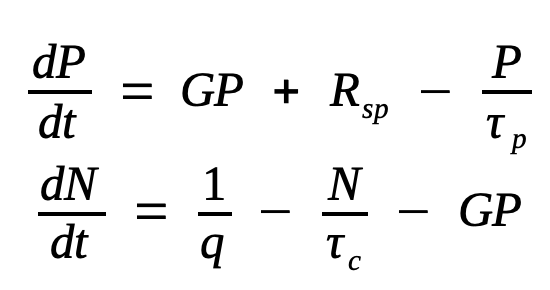where the net rate of stimulated emission G is defined as

G = Γvggm = GN(N-N0)

and Rsp is the rate of spontaneous emission into the lasing mode. Note that

Rsp is much smaller than the total spontaneous-emission rate. The reason is that spontaneous emission occurs in all directions over a wide spectral range (40-50 nm) but only a small fraction of it, propagating along the cavity axis and emitted at the laser frequency, actually contributes to the rate equation. In fact, Rsp and G are related as Rsp = NspG, where Nsp is the spontaneous emission factor introduced earlier and is about 2 for semiconductor lasers. The variable N represents the number of electrons rather than the carrier density; the two are related by the active volume V. In the equation, vg is the group velocity, Γ is the confinement factor, and gm is the material gain at the mode frequency. G varies linearly with N with GN = Γvgσg/V and N0 = NTV.

The last term in the first equation takes into account the loss of photons inside the cavity. The parameter τp is referred to as the photon lifetime. It is related to the cavity loss αcav introduced earlier as

τp-1 = vgαcav = vgmir + αint)

The three terms in the second rate equation indicates the rates at which electrons are created or destroyed inside the active region. The carrier lifetime τc includes the loss of electrons owing to both spontaneous emission and nonradiative recombination.

The P-I curve characterizes the emission properties of a semiconductor laser, as it indicates not in the threshold level but also the current that needs to be applied to obtain a certain amount of power. The figure below shows the P-I curve of a 1.3-µm InGaAsP laser at temperatures in the range 10-130ºC. At room temperature, the threshold is reached near 20 mA, and the laser can emit 10 mW of output power from each facet at 100 mA of applied current. The laser performance degrades at high temperatures. The threshold current is found to increase exponentially with temperature, that is

Ith(T) = I0exp(T/T0)

where I0 is a constant and T0 is a characteristic temperature often used to express the temperature sensitivity of threshold current. For InGaAsP lasers T0 is typically in the range 50-70 K. By contrast, T0 exceeds 120 K for GaAs laser. Because of the temperature sensitivity of InGaAsP lasers, it is often necessary to control their temperature through a built-in thermoelectric cooler.The rate equations can be used to understand most of the features seen in the figure above. In the case of CW operation at a constant current I, the time derivatives in the rate equations above can be set to zero. The solution takes a particularly simple form if spontaneous emission is neglected by setting Rsp = 0. For currents such that p < 1, P = 0 and NτcI/q. The threshold is reached at a current for which p = 1. The carrier population is then clamped to the threshold value Nth = N0 + (GNτp)-1. The threshold current is given by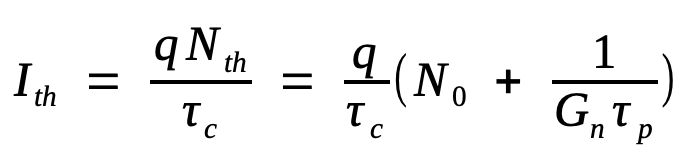For I > Ith, the photon number P increases linearly with I as

P = (τp/q)(IIth)

The emitted power Pe is related to P by the relation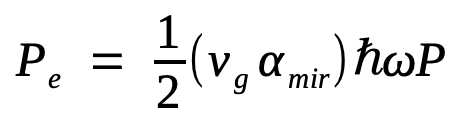The derivation of this equation is intuitively obvious if we note that vgαmir is the rate at which photons of energy ħω escape from the two facets. The factor of 1/2 makes Pe the power emitted from each facet for a FP laser with equal facet reflectivities. For FP lasers with coated facets or for DFB lasers, this equation needs to be suitably modified. The emitted power is given by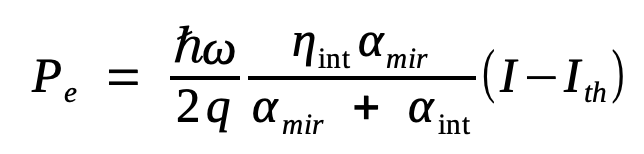where the internal quantum efficiency ηint is introduced phenomenologically to indicate the fraction of injected electrons that is converted into photons through stimulated emission. In the above-threshold regime, ηint is almost 100% for most semiconductor lasers.

A quantity of practical interest is the slope of the P-I curve for I > Ith; it is called the slope efficiency and is defined asThe quantity ηd is called the differential quantum efficiency, as it is a measure of the efficiency with which light output increases with an increase in the injected current.  One can define the external quantum efficiency ηext asηext and  ηd are related by

ηext = ηd(1 - Ith/I)

Generally,  ηext <  ηd but becomes nearly the same for I >> Ith. Similar to the case of LEDs, one can define the total quantum efficiency (or wall-plug efficiency) as ηtot = 2Pe/(V0I), where V0 is the applied voltage. It is related to ηext aswhere Eg is the bandgap energy. Generally, ηtot < ηext as the applied voltage exceeds Eg/q. For GaAs lasers, ηd can exceed 80% and ηtot can approach 50%. The InGaAsP lasers are less efficient with η≈ 50% and ηtot ~ 20%.

The exponential increase in the threshold current with temperature can be understood from the Ith equation above. The carrier lifetime τc is generally N-dependent because of Auger recombination and decreases with N as N2. The rate of Auger recombination increases exponentially with temperature and is responsible for the temperature sensitivity of InGaAsP lasers. The P-I curve also shows that the slope efficiency decreases with an increase in the output power (bending of the P-I curves). This decrease can be attributed to junction heating occurring under CW (continuous wave) operation. It can also result from an increase in internal loss or current leakage at high operating powers. Despite these problems, DFB lasers emitting >100 mW of power at room temperature in the 1.55-µm spectral region were fabricated by 1996 using a strained multiquantum-well (MQW) design. Such lasers exhibited <10 mW threshold current at 20ºC and emitted ~20 mW of power at 100ºC while maintaining a MSR of >40 dB. By 2003, DFB lasers capable of delivering more than 200 mW of power with a wavelength stability of <3 pm became available.

#### 2. Modulation Bandwidth

Output power of a DFB laser can be modulated directly if the applied current varies with time. The question is how fast this current can be modulated before the laser fails to respond to current variations. The modulation response of semiconductor lasers is studies by solving the rate equations with a time-dependent current of the form

I(t) = Ib + Imfp(t)

where Ib is the bias current, Im is the current, and fp(t) represents the shape of the current pulse.

Two changes are necessary for a realistic description. First the gain G must be modified to become

G = GN(N - N0)(1 - εNLP)

where εNL is a nonlinear-gain parameter that leads to a slight reduction in G as P increases. The physical mechanism behind this reduction can be attributed to several phenomena, such as spatial hole burning, spectral hole burning, carrier heating, and two-photon absorption. Typical values of εNL are ~10-7. This equation is valid for εNLP << 1. The factor 1 - εNLP should be replaced by (1+P/Ps)-b, where Ps is a material parameter, when the laser power exceeds far above 10 mW. The exponent b equals 1/2 for spectral hole burning but can vary over the range 0.2-1 because of the contribution of carrier heating.

The second change is related to an important property of semiconductor lasers. It turns out that whenever the optical gain changes as a result of changes in the carrier population N, the refractive index also changes. From a physical standpoint, amplitude modulation in semiconductor lasers is always accompanied by phase modulation because of carrier-induced changes in the mode index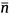. Phase modulation can be included through the equation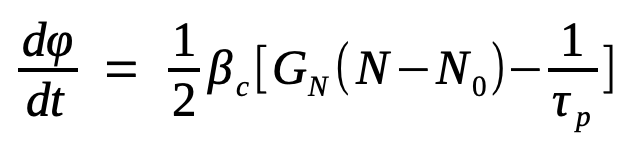where βc is the amplitude-phase coupling parameter, commonly called the linewidth enhancement factor, as it leads to an enhancement of the spectral width associated with a single longitudinal mode. Typical values of βc for InGaAsP lasers are in the range 4-8, depending on the operating wavelength. Lower values of βc occur in MQW lasers, especially for strained quantum wells.

In general, the nonlinear nature of the rate equations makes it necessary to solve them numerically. A useful analytic solution can be obtained for the case of small-signal modulation in which the laser is biased above threshold (Ib > Ith) and modulated such that Im << Ib - Ith. The rate equations can be linearized in that case and solved analytically, using the Fourier transform technique, for an arbitrary form of fp(t). The small-signal modulation bandwidth can be obtained by considering the response of semiconductor lasers to sinusoidal modulation at the frequency ωm so that fp(t) = sin(ωmt). The laser output is also modulated sinusoidally. The general solution of the rate equations is given by

P(t) = Pb + |pm| sin(ωmt + θm)

N(t) = Nb + |nm| sin(ωmt + ψm)

where Pb and Nb are the steady-state values at the bias current Ib|pm| and |nm| are small changes occurring because of current modulation, and θm and ψm govern the phase lag associated with the small-signal modulation. In particular, pm ≡ |pm| exp(iθm) is given by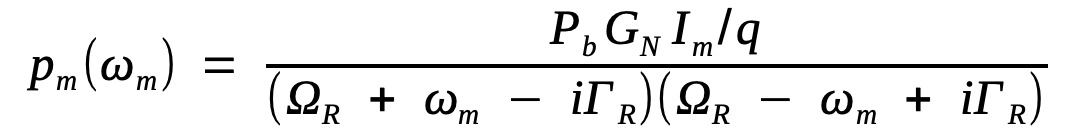where

ΩR = [GGNPb - (ΓP - ΓN)2/4]1/2,     ΓR = (ΓP + ΓN)/2

ΓP = Rsp/Pb + εNLGPb,   ΓN = τc-1 + GNPb

ΩR and ΓR are the frequency and the damping rate of relaxation oscillations. These two parameters play an important role in governing the dynamic response of semiconductor lasers. In particularly, the efficiency is reduced when the modulation frequency exceeds ΩR by a large amount.

It is common to introduce a power-transfer function as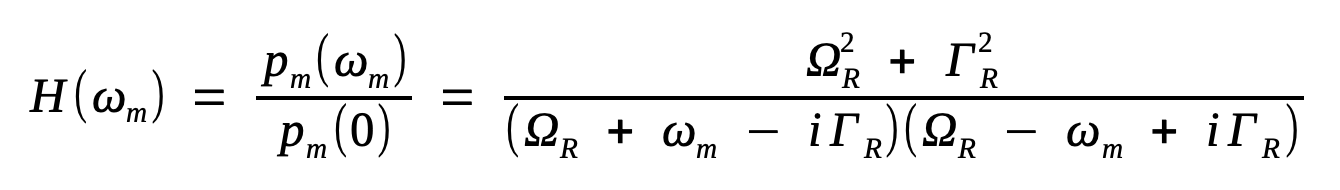The modulation response is flat [H(ωm 1] for frequencies such that ωm << ΩR, peaks at ωm = ΩR, and then drops sharply for ωm >> ΩR. These features are observed experimentally for all semiconductor lasers. The following figure shows the modulation response of a 1.55-µm DFB laser at several bias levels.The 3-dB modulation bandwidth, f3 dB, is defined as the frequency at which |H(ωm)| is reduced by 3 dB (by a factor of 2) compared with its direct-current (dc) value. The equation above provides the following analytic expression for f3 dB: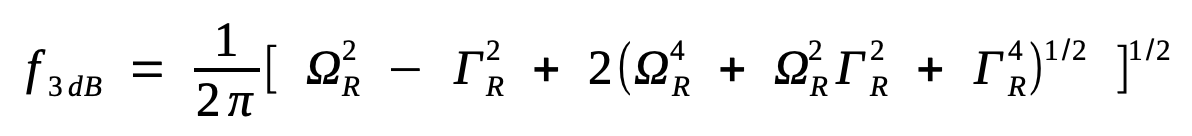For most laser, ΓR << ΩR, and f3 dB can be approximated by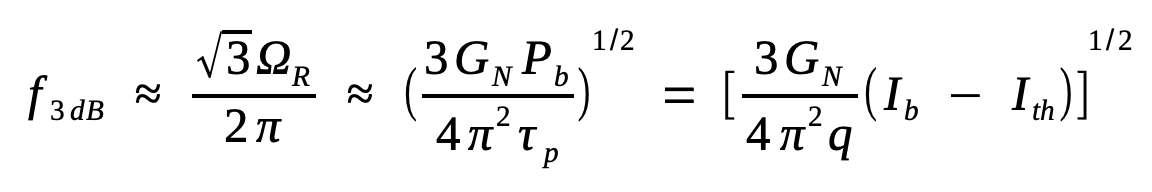where ΩR was approximated by (GGNPb)1/2 and G was replaced by 1/τp since gain equals loss in the above-threshold regime. The last expression was obtained at the bias level.

This equation provides a remarkably simple expression for the modulation bandwidth. It shows that f3 dB increases with an increase in the bias level as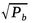or as (Ib - Ith)1/2. This square-root dependence has been verified for many DFB lasers exhibiting a modulation bandwidth of up to 30 GHz. The following figure shows how f3 dB can be increased to 24 GHz for a DFB laser by biasing it at 80 mA. A modulation bandwidth of 25 GHz was realized in 1994 for a packaged 1.55-µm InGaAsP laser specifically designed for high-speed response. The injection-locking technique is sometimes used to improve the modulation response of DFB lasers.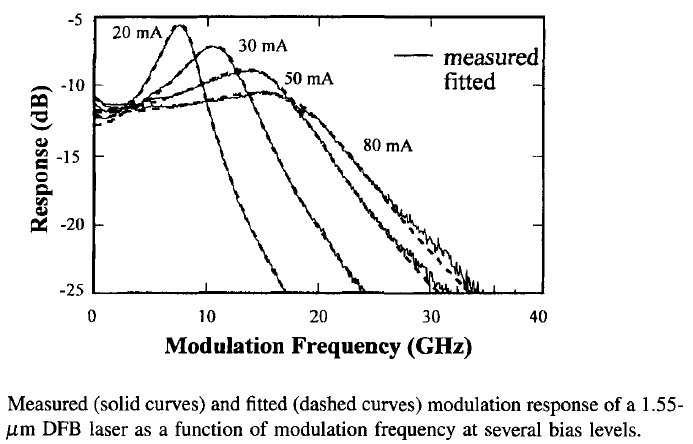#### 3. Relative Intensity Noise

The output of a semiconductor laser exhibits fluctuations in its intensity, phase, and frequency even when the laser is biased at a constant current with negligible current fluctuations. The two fundamental noise mechanisms are spontaneous emission and electron-hole recombination (shot noise).  Noise in semiconductor lasers is dominated by spontaneous emission. Each spontaneously emitted photon adds to the coherent field (established by stimulated emission) a small field component whose phase is random, and thus perturbs both the amplitude and phase in a random manner. Moreover, such spontaneous-emission events occur randomly at a high rate (~1012 s-1) because of a relatively large value of Rsp in semiconductor lasers. The net result is that the intensity and the phase of the emitted light exhibit fluctuations over a time scale as short as 100 ps. Intensity fluctuations lead to a limited signal-to-noise ratio (SNR), whereas phase fluctuations lead to a finite spectral linewidth when semiconductor lasers are operated at a constant current. Since such fluctuations can affect the performance of lightwave systems, it is important to estimate their magnitude.

The rate equations can be used to study laser noise by adding a noise term, known as the Langevin force, to each of them. The rate equations then become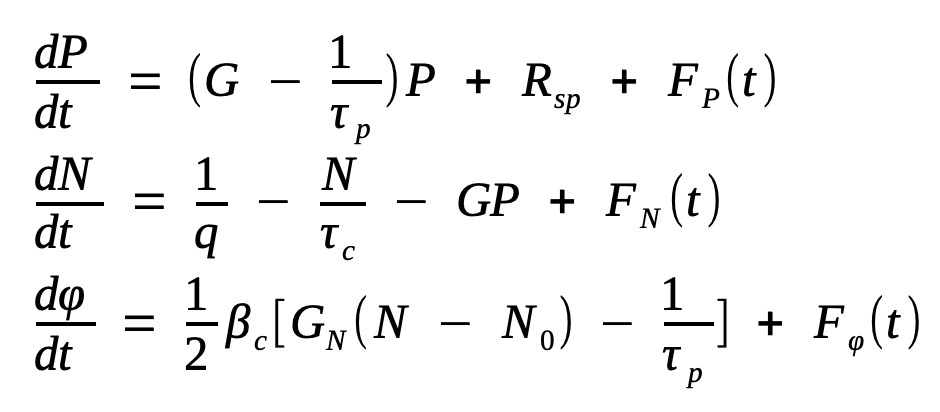where FP(t), FN(t), and Fφ(t) are the Langevin forces. They are assumed to be Gaussian random processes with zero mean and to have a correlation function of the form (the Markovian approximation)

<Fi(t)Fj(t')> = 2Dijδ(t-t')

where i, j = P, N, or φ, angle brackets denote the ensemble average, and Dij is called the diffusion coefficient. The dominant contribution to laser noise comes from only two diffusion coefficients DPP = RspP and Dφφ = Rsp/4P; others can be assumed to be nearly zero.

The intensity-autocorrelation function is defined aswhereis the average value and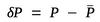represents a small fluctuation. The Fourier transform of Cpp(τ) is known as the relative-intensity-noise (RIN) spectrum and is given by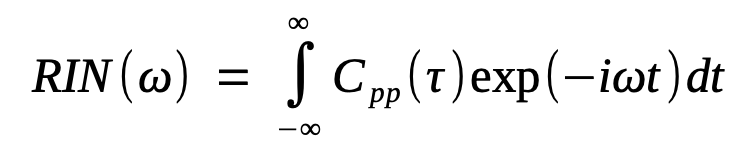The RIN is given approximately by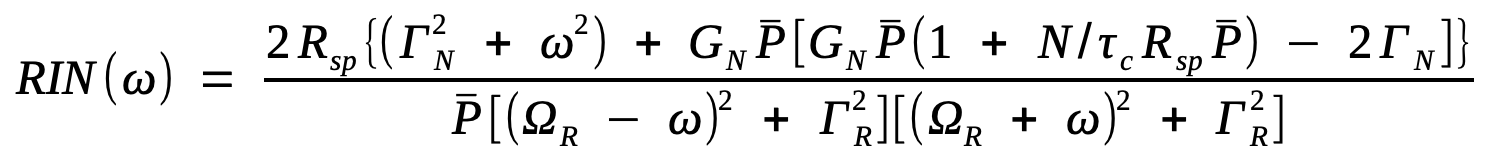where ΩR and ΓR are the frequency and the damping rate of relaxation oscillations.

The following figure shows the calculated RIN spectra at several power levels for a typical 1.55-µm InGaAsP laser.The RIN is considerably enhanced near the relaxation-oscillation frequency ΩR but decreases rapidly for ω >> ΩR, since the laser is not able to respond to fluctuations at such high frequencies. In essence, the semiconductor laser acts as a bandpass filter of bandwidth ΩR to spontaneous-emission fluctuations. At a given frequency, RIN decreases with an increase in the laser power as P-3 at low powers, but this behavior changes to P-1 dependence at high powers.

The autocorrelation function Cpp(τ) is calculated using the two equations above. The calculation shows that Cpp(τ) is follows relaxation oscillations and approaches zero for τ > ΓR-1. This behavior indicates that the intensity fluctuations do not remain correlated for times longer than the damping time of relaxation oscillations. The quantity of practical interest is the SNR defined as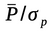, where σp is the root-mean-square (RMS) noise. From the last equations, SNR = |Cpp(τ)|-1/2. At power  levels above a few milliwatts, the SNR exceeds 20 dB and improves linearly with the power as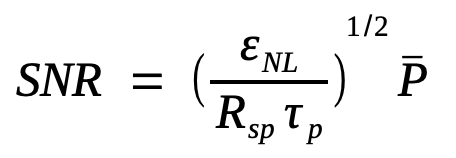The presence of εNL indicates that the nonlinear form of the gain plays a crucial role. This form needs to be modified at high powers. Indeed, a more accurate treatment shows that the SNR eventually saturates at a value of about 30 dB and becomes power-independent.

So far, the laser has been assumed to oscillate in a single longitudinal mode. In practice, even DFB lasers are accompanied by one or more side modes. Even though side modes remain suppressed by more than 20 dB on the basis of the average power, their presence can affect the RIN significantly. In particular, the main and side modes can fluctuate in such a way that individual modes exhibit large intensity fluctuations, but the total intensity remains relatively constant. This phenomenon is called mode-partition noise (MPN) and occurs due to an anticorrelation between the main and side modes. It manifests through the enhancement of RIN for the main mode by 20 dB or more in the low-frequency range 0-1 GHz; the exact value of the enhancement factor depends on the MSR. In the case of a VCSEL, the MPN involves two transverse modes. In the absence of fiber dispersion, MPN would be harmless for optical communication systems, as all modes would remain synchronized during transmission and detection. However, in practice all modes do not arrive simultaneously at the receiver because they travel at slightly different speeds. Such a desynchronization not only degrades the SNR of the received signal but also leads to intersymbol interference.

#### 4. Spectral Linewidth

The spectrum of emitted light is related to the field-autocorrelation function ΓEE(τ) through a Fourier-transform relation, that is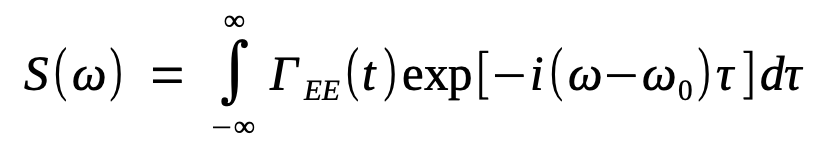where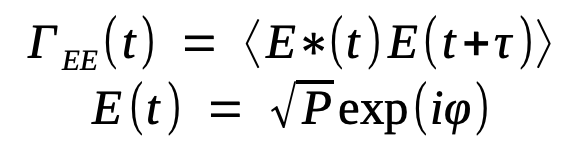E(t) is the optical field. If intensity fluctuations are neglected, ΓEE(t) is given bywhere the phase fluctuation Δφ(τ) = φ(t+τ) - φ(t) is taken to be a Gaussian random process. The phase variance〈Δφ2(τ)〉can be calculated by linearizing equations and solving the resulting set of linear equations. The result iswhere b = Ω/ (ΩR2 + ΓR2)1/2 and δ = tan-1ΓR/Ω).

The spectrum is obtained by these equations. It is found to consist of a dominant central peak located at ω0 and multiple satellite peaks located at ω = ω0 ±mΩwhere m is an integer. The amplitude of satellite peaks is typically less than 1% of that of the central peak. The physical origin of the satellite peaks is related to relaxation oscillations, which are responsible for the term proportional to b in the equation above. If this term is neglected, the autocorrelation function ΓEE(τ) decays exponentially with τ. The integral in the equation can then be performed analytically, and the spectrum is found to be Lorentzian. The spectral linewidth Δν is defined as the full-width at half-maximum (FWHM) of this Lorentzian line and is given by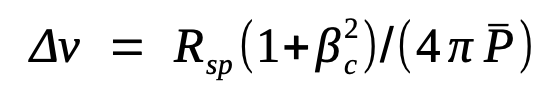where b = 1 was assumed as ΓR << ΩR under typical operating conditions. The linewidth is enhanced by a factor of 1+βc2 as a result of the amplitude-phase coupling governed by βcβc is called the linewidth enhancement factor for this reason.

The spectral linewidth equation above shows that Δν should decrease aswith an increase in the laser power. Such an inverse dependence is observed experimentally at low power levels (<10 mW) for most semiconductor lasers. However, often the linewidth is found to saturate to a value in the range of 1-10 MHz at a power level above 10 mW. The figure below shows such linewidth-saturation behavior for several 1.55-µm DFB lasers. It also shows that the linewidth can be reduced considerably by using a MQW design for the DFB laser. The reduction is due to a small value of the parameter βc realized by such a design.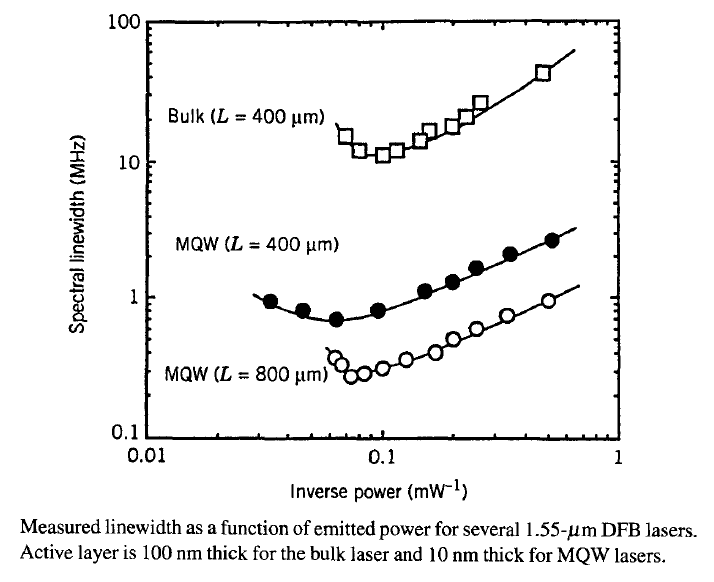The linewidth can also be reduced by increasing the cavity length L, since Rsp decreases and P increases at a given output power as L is increased. Although not obvious from the equation, Δν can be shown to vary as L-2 when the length dependence of Rsp and P is incorporated. As seen in the figure above, Δν is reduced by about a factor of 4 when the cavity length is doubled. The 800-µm-long MQW-DFB laser is found to exhibit a linewidth as small as 270 kHz at a power output of 13.5 mW. It is further reduced in strained MQW lasers because of relatively low values of βc, and a value of about 100 kHz has been measured in lasers with βc ≈ 1. It should be stressed, however, that the linewidth of most DFB lasers is typically 5-10 MHz when operating at a power level of 10 mW. The figure above shows that as the laser power increases, the linewidth not only saturates but also begins to rebroaden. Several mechanisms such as current fluctuations, 1/f noise, nonlinear gain and index changes, and interaction with weak side modes have been invoked to explain this saturation. The linewidth of most DFB lasers is small enough that it is not a limiting factor for lightwave systems.